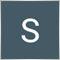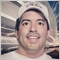# Expression as no effect14

This EA uses two signal to open buy order, one from custom indicator(Bb019)  and the other signal from crossing of moving average.

To open a trade custom indicator should give us first signal and confirmation of this signal is made by crossing of moving average

When running the following code I'm getting and error ( Expressing as no effect)

Whats the problem with this mql4 code?

for((iCustom(NULL, PERIOD_CURRENT, "Bb019", false, 5.0, 13.0, 34.0, 1, 8, 13, 1, 1, 175, 4, 0) != 0); Cross(1, iMA(NULL, PERIOD_CURRENT, 5, 0, MODE_LWMA, PRICE_CLOSE, 0) > iMA(NULL, PERIOD_CURRENT, 6, 0, MODE_SMA, PRICE_CLOSE, 0))

; )
{
RefreshRates();
{
if(ticket <= 0) return;
}
}Moderator
20585

I cannot make any sense of what you are trying to do with the for()8830

majoh12:

This EA uses two signal to open buy order, one from custom indicator(Bb019)  and the other signal from crossing of moving average.

To open a trade custom indicator should give us first signal and confirmation of this signal is made by crossing of moving average

When running the following code I'm getting and error ( Expressing as no effect)

Whats the problem with this mql4 code?

for((iCustom(NULL, PERIOD_CURRENT, "Bb019", false, 5.0, 13.0, 34.0, 1, 8, 13, 1, 1, 175, 4, 0) != 0); Cross(1, iMA(NULL, PERIOD_CURRENT, 5, 0, MODE_LWMA, PRICE_CLOSE, 0) > iMA(NULL, PERIOD_CURRENT, 6, 0, MODE_SMA, PRICE_CLOSE, 0))

; )
{
RefreshRates();
{
if(ticket <= 0) return;
}
}

The problem is that you use an expression which has no effect :) and as Keith wrote 'I cannot make any sense of what you are trying to do with the for()' i have to agree. That for() thing is totally wrong approach and it all seems like a mess.Beside all this use alt+s when inserting code here..it looks like a total mess everything9

Instead of using   for() you were supposed to use if()

if ((iCustom(NULL, PERIOD_CURRENT, "Bb019", false, 5.0, 13.0, 34.0, 1, 8, 13, 1, 1, 175, 4, 0) != 0); Cross(1, iMA(NULL, PERIOD_CURRENT, 5, 0, MODE_LWMA, PRICE_CLOSE, 0) > iMA(NULL, PERIOD_CURRENT, 6, 0, MODE_SMA, PRICE_CLOSE, 0))

; )
{
RefreshRates();
{
if(ticket <= 0) return;
}
}20250

Please edit your (original) post and use the CODE button (Alt-S)! (For large amounts of code, attach it.)
General rules and best pratices of the Forum. - General - MQL5 programming forum
Messages Editor167

Swahili fx:

Instead of using   for() you were supposed to use if()

if ((iCustom(NULL, PERIOD_CURRENT, "Bb019", false, 5.0, 13.0, 34.0, 1, 8, 13, 1, 1, 175, 4, 0) != 0); Cross(1, iMA(NULL, PERIOD_CURRENT, 5, 0, MODE_LWMA, PRICE_CLOSE, 0) > iMA(NULL, PERIOD_CURRENT, 6, 0, MODE_SMA, PRICE_CLOSE, 0))

; )
{
RefreshRates();
{
if(ticket <= 0) return;
}
}

The code is wrong, it is not following C Language Syntax.

You cannot either use for() or if() and terminate the logical operation using ";"

By using ";"  you are terminating the logical operation and it cannot continue on the lines of code below it.

if() syntax requires some code to be executed

eg:

```if ((x) != 0) {

execute_me();

}

```

or

```if ((x) != 0) execute_me();
```

But never

```if ((x) != 0) ;
{
execute_me();
}

```

Your condition below is never executed

```if ((iCustom(NULL, PERIOD_CURRENT, "Bb019", false, 5.0, 13.0, 34.0, 1, 8, 13, 1, 1, 175, 4, 0) != 0); Cross(1, iMA(NULL, PERIOD_CURRENT, 5, 0, MODE_LWMA, PRICE_CLOSE, 0) > iMA(NULL, PERIOD_CURRENT, 6, 0, MODE_SMA, PRICE_CLOSE, 0))

// --- the same a above, indented:
if ((iCustom(NULL, PERIOD_CURRENT, "Bb019", false, 5.0, 13.0, 34.0, 1, 8, 13, 1, 1, 175, 4, 0) != 0);
{
Cross(1, iMA(NULL, PERIOD_CURRENT, 5, 0, MODE_LWMA, PRICE_CLOSE, 0) > iMA(NULL, PERIOD_CURRENT, 6, 0, MODE_SMA, PRICE_CLOSE, 0))
}

Wrong syntax```

You need to remove the ";"

Considering you want to compare 2 things, the code would be:

```if (
(iCustom(NULL, PERIOD_CURRENT, "Bb019", false, 5.0, 13.0, 34.0, 1, 8, 13, 1, 1, 175, 4, 0) != 0)
&& (Cross(1, (iMA(NULL, PERIOD_CURRENT, 5, 0, MODE_LWMA, PRICE_CLOSE, 0)) > (iMA(NULL, PERIOD_CURRENT, 6, 0, MODE_SMA, PRICE_CLOSE, 0)) ))
)
{
RefreshRates();

// put the rest of the code here to be executed...

}

```

The above is an exemple of syntax, adapt it for the correct cross() function parameters as you need it to do it.